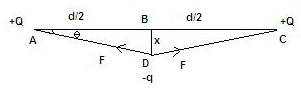# Two positive charges +Q are held fixed a distance d apart. A particle of negative charge -q and mass m is placed midway between them, then is given a small displacement perpendicular to the line joining them and released. Show that the particle describes simple harmonic motion

12 years agohere, net restoring force , F' =-2Fsiny                     y=thita

so,  F=kQqsin^2 y/x^2

thus,  F'=-2kQqsin^3 y/x^2

but , as siny is very small siny=y

so, F'=-2kQqy^3/x^2

since , y=2x/d

so , F'=-16kQqx/d^3

or, F'=-(16kQq/d^3) x

or, a'=-(16kQq/md^3) x

thus acceleration is proportional to -x(displacement) hence the charge -q

will perform simple harmonic motion with angular frequency (16kQq/md^3)^1/2Yash Chourasiya
3 years ago
Hello Studenthere, net restoring force , F' =-2Fsinθ

Now, F = kQq/(x/sinθ)2

so, F = kQqsin2θ/x2

thus, F' = -2kQqsin3θ/x2

but , as sinθ is very small sinθ =θ

so, F' = -2kQqθ3/x2

since , θ = 2x/d

so , F' = -16kQqx/d3

or, F' = -(16kQq/d3) x

or, a' = -(16kQq/md3) x

thus acceleration is proportional to -x(displacement) hence the charge -q

will perform simple harmonic motion with angular frequency √(16kQq/md3)